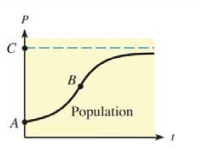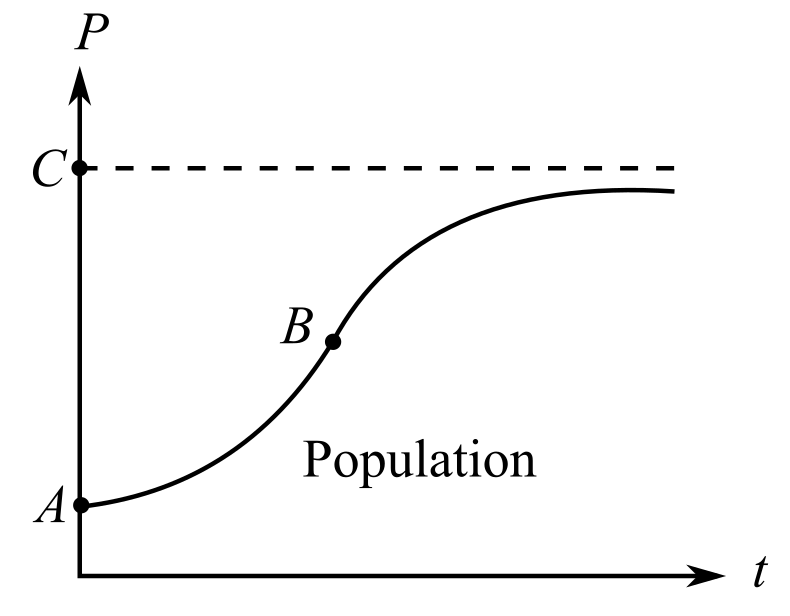Chapter 10.2, Problem 32E### Mathematical Applications for the ...

11th Edition
Ronald J. Harshbarger + 1 other
ISBN: 9781305108042

#### Solutions

Chapter
Section### Mathematical Applications for the ...

11th Edition
Ronald J. Harshbarger + 1 other
ISBN: 9781305108042
Textbook Problem

# Population growth The figure shows the growth of a population as a function of time.(a) If P represents the population and t represents the time, write a mathematical symbol that represents the rate of change (growth rate) of the population with respect to time.(b) Which of A, B, and C corresponds to the point at which the growth rate attains its maximum?(c) Which of A, B, and C corresponds to the upper limit of population?

(a)

To determine

To calculate: The mathematical symbol for rate of change of population with respect to time if P represents the population and t represents time.

Explanation

Given Information:

The P represents the population and t represents time.

Calculation:

Consider P represents the population and t represents time.

Recall that rate of change of a function with respect to an independent variable is the derivative of that function with respect to that variable.

Thus, rate of change of population with respect to time is the derivative of the function P(t) with respect to t.

Differentiate P(t) with respect to t

(b)

To determine

To calculate: The point at which the growth rate is maximum if P represents the population and t represents time and the graph is(c)

To determine

The point corresponds to the upper limit of worker population where the figure represents graph of population as a function of time on the job,### Still sussing out bartleby?

Check out a sample textbook solution.

See a sample solution

#### The Solution to Your Study Problems

Bartleby provides explanations to thousands of textbook problems written by our experts, many with advanced degrees!

Get Started